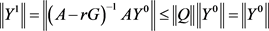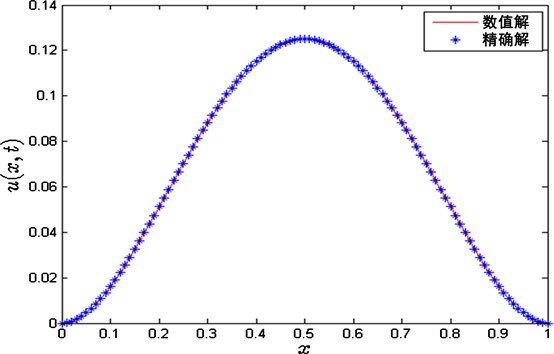﻿ 空间分布阶时间分数阶扩散方程的有限体积法

空间分布阶时间分数阶扩散方程的有限体积法Finite Volume Method for a Space Distributed-Order Time-Fractional Diffusion Equation

Abstract: In this paper, the space distributed-order time-fractional diffusion equation is considered. We propose a finite volume method to solve the considered equation. Firstly, we use the mid-point quadrature rule to transform the space distributed-order term into a multi-term fractional term, and the multi-term fractional equation is discretized by the finite volume. For the time-fractional derivative, we apply the finite difference method. We prove that the iterative scheme is uncondi-tionally stable and convergent. A numerical example is presented to verify the effectiveness of the proposed method.

1. 引言

$\frac{{\partial }^{\beta }u\left(x,t\right)}{\partial {t}^{\beta }}={\int }_{1}^{2}P\left(\alpha \right)\frac{{\partial }^{\alpha }u\left(x,t\right)}{\partial {|x|}^{\alpha }}\text{d}\alpha +f\left(x,t\right),\text{\hspace{0.17em}}\left(x,t\right)\in \left(0,L\right)×\left(0,T\right)$ , (1)

$f\left(x,t\right)$ 是源项， $P\left(\alpha \right)$ 是满足下面条件的非负权函数：

$P\left(\alpha \right)\ge 0$ , $P\left(\alpha \right)\overline{)\equiv }0$ , $\alpha \in \left(1,2\right)$ , $0<{\int }_{1}^{2}P\left(\alpha \right)\text{d}\alpha <\infty$ .

l和K是正的常数， ${A}_{1}>0$${A}_{2}>0$$0<{\alpha }_{1}<{\alpha }_{2}\le 2$$\frac{{\partial }^{\beta }u\left(x,t\right)}{\partial {t}^{\beta }}$ 定义为Caputo分数阶导数：

$\frac{{\partial }^{\beta }u\left(x,t\right)}{\partial {t}^{\beta }}=\frac{1}{\Gamma \left(1-\beta \right)}{\int }_{0}^{t}\frac{\partial u\left(x,\xi \right)}{\partial \xi }\frac{\text{d}\xi }{\left(t-\xi \right)}，\text{\hspace{0.17em}}0<\beta <1$ .

$\frac{{\partial }^{\alpha }u\left(x,t\right)}{\partial {|x|}^{\alpha }}$ 是Riesz分数阶导数，定义为：

$\frac{{\partial }^{\alpha }u\left(x,t\right)}{\partial {|x|}^{\alpha }}=-\frac{1}{2\mathrm{cos}\left(\alpha \text{π}/2\right)}\left(\frac{{\partial }^{\alpha }u\left(x,t\right)}{\partial {x}^{\alpha }}+\frac{{\partial }^{\alpha }u\left(x,t\right)}{\partial {\left(-x\right)}^{\alpha }}\right),\text{\hspace{0.17em}}1<\alpha \le 2$ .

2. 有限体积法求解空间分布阶时间分数阶扩散方程的Crank-Nicolson迭代格式

${t}_{n}=n\tau$$t=0,\text{​}\text{ }1,2,\cdots ,N$${x}_{i}=ih$$i=0,1,2,\cdots ,M$ 离散区间 $\left[0,L\right]×\left[0,T\right]$ ，其中 $h=L/M$$\tau =T/N$

$\begin{array}{c}\frac{{\partial }^{\beta }u\left(x,{t}_{n}\right)}{\partial {t}^{\beta }}=\frac{1}{\Gamma \left(1-\beta \right)}\underset{j=0}{\overset{n-1}{\sum }}{\int }_{j\tau }^{\left(j+1\right)\tau }\frac{\partial u\left(x,\xi \right)}{\partial \xi }\frac{\text{d}\xi }{{\left({t}_{n}-\xi \right)}^{\beta }}\\ =\frac{1}{\Gamma \left(1-\beta \right)}\underset{j=0}{\overset{n-1}{\sum }}\frac{u\left(x,{t}_{j+1}\right)-u\left(x,{t}_{j}\right)}{\tau }{\int }_{j\tau }^{\left(j+1\right)\tau }\frac{\text{d}\xi }{{\left({t}_{n}-\xi \right)}^{\beta }}+ο\left({\tau }^{2-\beta }\right)\\ =\frac{{\tau }^{1-\beta }}{\Gamma \left(2-\beta \right)}\underset{j=0}{\overset{n-1}{\sum }}\frac{u\left(x,{t}_{n-j}\right)-u\left(x,{t}_{n-j-1}\right)}{\tau }\left[{\left(j+1\right)}^{1-\beta }-{j}^{1-\beta }\right]+ο\left({\tau }^{2-\beta }\right)\\ =a\left[u\left(x,{t}_{n}\right)-u\left(x,{t}_{n-1}\right)\right]+a\underset{j=1}{\overset{n-1}{\sum }}\left[u\left(x,{t}_{n-j}\right)-u\left(x,{t}_{n-j-1}\right)\right]{b}_{j}+ο\left({\tau }^{2-\beta }\right)\end{array}$ (4)

${\int }_{1}^{2}P\left(\alpha \right)\frac{{\partial }^{\alpha }u\left(x,t\right)}{\partial {|x|}^{\alpha }}\text{d}\alpha ={\int }_{{1}^{+}}^{2}P\left(\alpha \right)\frac{{\partial }^{\alpha }u\left(x,t\right)}{\partial {|x|}^{\alpha }}\text{d}\alpha$ . (5)

$\begin{array}{l}{\int }_{{1}^{+}}^{2}P\left(\alpha \right)\frac{{\partial }^{\alpha }u\left(x,t\right)}{\partial {|x|}^{\alpha }}\text{d}\alpha \\ =\underset{k=1}{\overset{S}{\sum }}P\left({\alpha }_{k}\right)\frac{{\partial }^{{\alpha }_{k}}u\left(x,t\right)}{\partial {|x|}^{{\alpha }_{k}}}\Delta {\xi }_{k}+ο\left({\sigma }^{2}\right)\end{array}$ . (6)

$\begin{array}{l}a\left[u\left(x,{t}_{n}\right)-u\left(x,{t}_{n-1}\right)\right]+a\underset{j=1}{\overset{n-1}{\sum }}{b}_{j}\left[u\left(x,{t}_{n-j}\right)-u\left(x,{t}_{n-j-1}\right)\right]\\ =\sigma \underset{k=1}{\overset{S}{\sum }}P\left({\alpha }_{k}\right)\frac{{\partial }^{{\alpha }_{k}}u\left(x,{t}_{n}\right)}{\partial {|x|}^{{\alpha }_{k}}}+f\left(x,{t}_{n}\right)+ο\left({\tau }^{2-\beta }+{\sigma }^{2}\right)\\ =\sigma \underset{k=1}{\overset{S}{\sum }}{a}_{k}P\left({\alpha }_{k}\right)\frac{\partial }{\partial x}\left[\frac{{\partial }^{{\gamma }_{k}}u\left(x,{t}_{n}\right)}{\partial {x}^{{\gamma }_{k}}}-\frac{{\partial }^{{\gamma }_{k}}u\left(x,{t}_{n}\right)}{\partial {\left(-x\right)}^{{\gamma }_{k}}}\right]+f\left(x,{t}_{n}\right)+ο\left({\tau }^{2-\beta }+{\sigma }^{2}\right)\end{array}$ (7)

$\begin{array}{l}a{\int }_{{x}_{i-\frac{1}{2}}}^{{x}_{i+\frac{1}{2}}}u\left(x,{t}_{n}\right)\text{d}x-\sigma \underset{k=1}{\overset{S}{\sum }}{a}_{k}P\left({\alpha }_{k}\right){\left[\frac{{\partial }^{{\gamma }_{k}}u\left(x,{t}_{n}\right)}{\partial {x}^{{\gamma }_{k}}}-\frac{{\partial }^{{\gamma }_{k}}u\left(x,{t}_{n}\right)}{\partial {\left(-x\right)}^{{\gamma }_{k}}}\right]}_{{x}_{i-\frac{1}{2}}}^{{x}_{i+\frac{1}{2}}}\\ =a{\int }_{{x}_{i-\frac{1}{2}}}^{{x}_{i+\frac{1}{2}}}u\left(x,{t}_{n-1}\right)\text{d}x-a\underset{j=1}{\overset{n-1}{\sum }}{b}_{j}{\int }_{{x}_{i-\frac{1}{2}}}^{{x}_{i+\frac{1}{2}}}\left[u\left(x,{t}_{n-j}\right)-u\left(x,{t}_{n-j-1}\right)\right]\text{d}x+{\int }_{{x}_{i-\frac{1}{2}}}^{{x}_{i+\frac{1}{2}}}f\left(x,{t}_{n}\right)\text{d}x+ο\left(h\left({\tau }^{2-\beta }+\sigma \right)\right)\end{array}$ (8)

${\varphi }_{i},0\le i\le M$${V}_{h}$ 的节点基函数。 ${\varphi }_{0},{\varphi }_{1},\cdots ,{\varphi }_{M}$ 表示为下面形式：

${\varphi }_{i}\left(x\right)=\left\{\begin{array}{l}\frac{x-{x}_{i-1}}{h},\text{ }x\in \left[{x}_{i-1},{x}_{i}\right]\\ \frac{{x}_{i+1}-x}{h},\text{ }x\in \left[{x}_{i},{x}_{i+1}\right],\text{ }i=1,2,\cdots ,M-1\\ 0,\text{\hspace{0.17em}}\text{ }\text{ }\text{ }\text{\hspace{0.17em}}其它\end{array}$${\varphi }_{0}\left(x\right)=\left\{\begin{array}{l}\frac{{x}_{1}-x}{h},\text{ }x\in \left[{x}_{0},{x}_{1}\right]\\ 0,\text{\hspace{0.17em}}\text{ }\text{ }\text{ }\text{\hspace{0.17em}}其它\end{array}$${\varphi }_{M}\left(x\right)=\left\{\begin{array}{l}\frac{x-{x}_{M-1}}{h},\text{ }x\in \left[{x}_{M-1},{x}_{M}\right]\\ 0,\text{\hspace{0.17em}}\text{ }\text{ }\text{ }\text{\hspace{0.17em}}\text{\hspace{0.17em}}\text{\hspace{0.17em}}\text{\hspace{0.17em}}其它\end{array}$

$\begin{array}{l}a\underset{m=1}{\overset{M-1}{\sum }}{u}_{m}^{n}{\int }_{{x}_{i-\frac{1}{2}}}^{{x}_{i+\frac{1}{2}}}{\varphi }_{m}\left(x\right)\text{d}x-\sigma \underset{m=1}{\overset{M-1}{\sum }}{u}_{m}^{n}\underset{k=1}{\overset{S}{\sum }}{a}_{k}P\left({\alpha }_{k}\right){\left[\frac{{\partial }^{{\gamma }_{k}}{\varphi }_{m}\left(x\right)}{\partial {x}^{{\gamma }_{k}}}-\frac{{\partial }^{{\gamma }_{k}}{\varphi }_{m}\left(x\right)}{\partial {\left(-x\right)}^{{\gamma }_{k}}}\right]}_{{x}_{i-\frac{1}{2}}}^{{x}_{i+\frac{1}{2}}}\\ =a\underset{m=1}{\overset{M-1}{\sum }}{u}_{m}^{n-1}{\int }_{{x}_{i-\frac{1}{2}}}^{{x}_{i+\frac{1}{2}}}{\varphi }_{m}\left(x\right)\text{d}x-a\underset{m=1}{\overset{M-1}{\sum }}\underset{j=1}{\overset{n-1}{\sum }}{b}_{j}{u}_{m}^{n-j}{\int }_{{x}_{i-\frac{1}{2}}}^{{x}_{i+\frac{1}{2}}}{\varphi }_{m}\left(x\right)\text{d}x+a\underset{m=1}{\overset{M-1}{\sum }}\underset{j=1}{\overset{n-1}{\sum }}{b}_{j}{u}_{m}^{n-j-1}{\int }_{{x}_{i-\frac{1}{2}}}^{{x}_{i+\frac{1}{2}}}{\varphi }_{m}\left(x\right)\text{d}x+{\int }_{{x}_{i-\frac{1}{2}}}^{{x}_{i+\frac{1}{2}}}f\left(x,{t}_{n}\right)\text{d}x\end{array}$ (9)

${\int }_{{x}_{i-\frac{1}{2}}}^{{x}_{i+\frac{1}{2}}}{\varphi }_{m}\left(x\right)\text{d}x=\left\{\begin{array}{l}h/8,\text{ }\text{\hspace{0.17em}}|i-m|=1\\ 3h/4,\text{ }i=m\\ 0,\text{ }\text{ }\text{\hspace{0.17em}}\text{\hspace{0.17em}}其它\end{array}$

$\frac{{\partial }^{{\gamma }_{k}}{\varphi }_{m}\left({x}_{i+1/2}\right)}{\partial {x}^{{\gamma }_{k}}}=\frac{1}{\Gamma \left(2-{\gamma }_{k}\right){h}^{{\gamma }_{k}}}\left\{\begin{array}{l}0,\text{ }\text{ }\text{ }\text{ }\text{ }\text{\hspace{0.17em}}\text{\hspace{0.17em}}\text{\hspace{0.17em}}m>i+1\\ {2}^{{\gamma }_{k}-1},\text{ }\text{ }\text{ }\text{ }\text{\hspace{0.17em}}m=i+1\\ {\left(3/2\right)}^{1-{\gamma }_{k}}-{2}^{{\gamma }_{k}},\text{\hspace{0.17em}}\text{\hspace{0.17em}}m=i\\ {c}_{i-m+1}^{k},\text{ }\text{ }\text{ }\text{\hspace{0.17em}}\text{\hspace{0.17em}}\text{\hspace{0.17em}}\text{ }m

$\frac{{\partial }^{{\gamma }_{k}}{\varphi }_{m}\left({x}_{i-1/2}\right)}{\partial {x}^{{\gamma }_{k}}}=\frac{1}{\Gamma \left(2-{\gamma }_{k}\right){h}^{{\gamma }_{k}}}\left\{\begin{array}{l}0,\text{ }\text{ }\text{ }\text{ }\text{ }\text{\hspace{0.17em}}\text{\hspace{0.17em}}m>i\\ {2}^{{\gamma }_{k}-1},\text{ }\text{ }\text{ }\text{ }\text{ }\text{ }m=i\\ {\left(3/2\right)}^{1-{\gamma }_{k}}-{2}^{{\gamma }_{k}},\text{ }\text{ }\text{ }\text{ }\text{ }m=i-1\\ {c}_{i-m}^{k},\text{ }\text{ }\text{ }\text{\hspace{0.17em}}\text{\hspace{0.17em}}\text{\hspace{0.17em}}\text{\hspace{0.17em}}\text{ }\text{ }m

$\frac{{\partial }^{{\gamma }_{k}}{\varphi }_{m}\left({x}_{i+1/2}\right)}{\partial {\left(-x\right)}^{{\gamma }_{k}}}=\frac{1}{\Gamma \left(2-{\gamma }_{k}\right){h}^{{\gamma }_{k}}}\left\{\begin{array}{l}{c}_{m-i}^{k},\text{ }\text{ }\text{ }\text{ }\text{\hspace{0.17em}}m>i+1\\ {\left(3/2\right)}^{1-{\gamma }_{k}}-{2}^{{\gamma }_{k}},m=i-1\\ {2}^{{\gamma }_{k}-1},\text{ }\text{ }\text{ }\text{ }m=i\\ 0,\text{ }\text{ }\text{ }\text{\hspace{0.17em}}\text{\hspace{0.17em}}\text{\hspace{0.17em}}\text{\hspace{0.17em}}\text{\hspace{0.17em}}\text{ }m

$\frac{{\partial }^{{\gamma }_{k}}{\varphi }_{m}\left({x}_{i-1/2}\right)}{\partial {\left(-x\right)}^{{\gamma }_{k}}}=\frac{1}{\Gamma \left(2-{\gamma }_{k}\right){h}^{{\gamma }_{k}}}\left\{\begin{array}{l}{c}_{m-i+1}^{k},\text{ }\text{ }\text{ }\text{\hspace{0.17em}}\text{​}\text{\hspace{0.17em}}\text{ }m>i\\ {\left(3/2\right)}^{1-{\gamma }_{k}}-{2}^{{\gamma }_{k}},\text{\hspace{0.17em}}m=i\\ {2}^{{\gamma }_{k}-1},\text{ }\text{ }\text{ }\text{ }m=i-1\\ 0,\text{ }\text{ }\text{ }\text{\hspace{0.17em}}\text{\hspace{0.17em}}\text{\hspace{0.17em}}\text{\hspace{0.17em}}\text{\hspace{0.17em}}\text{ }m

${G}_{1,im}=\sigma \underset{k=1}{\overset{S}{\sum }}{a}_{k}P\left({\alpha }_{k}\right)\left[\frac{{\partial }^{{\gamma }_{k}}{\varphi }_{m}\left({x}_{i+1/2}\right)}{\partial {x}^{{\gamma }_{k}}}-\frac{{\partial }^{{\gamma }_{k}}{\varphi }_{m}\left({x}_{i-1/2}\right)}{\partial {x}^{{\gamma }_{k}}}\right]$ , ${G}_{2,im}=\sigma \underset{k=1}{\overset{S}{\sum }}{a}_{k}P\left({\alpha }_{k}\right)\left[\frac{{\partial }^{{\gamma }_{k}}{\varphi }_{m}\left({x}_{i+1/2}\right)}{\partial {\left(-x\right)}^{{\gamma }_{k}}}-\frac{{\partial }^{{\gamma }_{k}}{\varphi }_{m}\left({x}_{i-1/2}\right)}{\partial {\left(-x\right)}^{{\gamma }_{k}}}\right]$ .

$a\frac{h}{8}\left({u}_{i-1}^{1}+6{u}_{i}^{1}+{u}_{i+1}^{1}\right)-\underset{m=1}{\overset{M-1}{\sum }}{u}_{m}^{1}{G}_{im}=a\frac{h}{8}\left({u}_{i-1}^{0}+6{u}_{i}^{0}+{u}_{i+1}^{0}\right)+{\left({F}^{1}\right)}_{i}$ , (10)

$n>1$ 时，(11)

$\left\{\begin{array}{l}\left(A-rG\right){U}^{1}=A{U}^{0}+rF{}^{1},\text{ }\text{ }\text{ }\text{ }\text{ }\text{ }\text{ }\text{ }\text{ }\text{ }\text{ }\text{ }\text{ }\text{ }\text{\hspace{0.17em}}\text{\hspace{0.17em}}\text{\hspace{0.17em}}\text{\hspace{0.17em}}\text{\hspace{0.17em}}\text{ }n=1\\ \left(A-rG\right){U}^{n}=A\left({d}_{1}{U}^{n-1}+{d}_{2}{U}^{n-2}+\cdots +{d}_{n-1}{U}^{1}+{b}_{n-1}{U}^{0}\right)+rF{}^{n},\text{\hspace{0.17em}}\text{\hspace{0.17em}}\text{ }n>1\end{array}$ (12)

3. 迭代方法的稳定性和收敛性

$|{G}_{ii}|>\underset{m=1,m\ne i}{\overset{M-1}{\sum }}|{G}_{im}|,i=1,2,\cdots ,M-1$ ，则G是严格对角占优的。

${B}_{im}=\left\{\begin{array}{l}r\sigma \underset{k=1}{\overset{S}{\sum }}\frac{{a}_{k}P\left({\alpha }_{k}\right)}{\Gamma \left(2-{\gamma }_{k}\right){h}^{{\gamma }_{k}}}\left({c}_{m-i}^{k}-{c}_{m-i+1}^{k}\right),\text{ }\text{ }\text{ }\text{ }\text{ }\text{ }\text{ }\text{ }\text{\hspace{0.17em}}\text{\hspace{0.17em}}m>i+1\\ \frac{h}{8}-r\sigma \underset{k=1}{\overset{S}{\sum }}\frac{{a}_{k}P\left({\alpha }_{k}\right)}{\Gamma \left(2-{\gamma }_{k}\right){h}^{{\gamma }_{k}}}\left[3{\left(\frac{1}{2}\right)}^{1-{\gamma }_{k}}-{\left(\frac{3}{2}\right)}^{1-{\gamma }_{k}}+{c}_{2}^{k}\right]，\text{ }\text{ }\text{\hspace{0.17em}}m=i+1\\ \frac{3h}{4}-r\sigma \underset{k=1}{\overset{S}{\sum }}\frac{{a}_{k}P\left({\alpha }_{k}\right)}{\Gamma \left(2-{\gamma }_{k}\right){h}^{{\gamma }_{k}}}2\left[{\left(\frac{3}{2}\right)}^{1-{\gamma }_{k}}-3{\left(\frac{1}{2}\right)}^{1-{\gamma }_{k}}\right],\text{ }\text{ }\text{ }m=i\\ \frac{h}{8}-r\sigma \underset{k=1}{\overset{S}{\sum }}\frac{{a}_{k}P\left({\alpha }_{k}\right)}{\Gamma \left(2-{\gamma }_{k}\right){h}^{{\gamma }_{k}}}\left[3{\left(\frac{1}{2}\right)}^{1-{\gamma }_{k}}-{\left(\frac{3}{2}\right)}^{1-{\gamma }_{k}}+{c}_{2}^{k}\right],\text{ }\text{ }\text{\hspace{0.17em}}m=i-1\\ r\sigma \underset{k=1}{\overset{S}{\sum }}\frac{{a}_{k}P\left({\alpha }_{k}\right)}{\Gamma \left(2-{\gamma }_{k}\right){h}^{{\gamma }_{k}}}\left({c}_{i-m}^{k}-{c}_{i-m+1}^{k}\right),\text{ }\text{ }\text{ }\text{ }\text{ }\text{ }\text{ }\text{ }\text{\hspace{0.17em}}\text{\hspace{0.17em}}m

$|{D}_{ii}|>\underset{m=1,m\ne i}{\overset{M-1}{\sum }}|{D}_{im}|,\text{\hspace{0.17em}}i=1,2,\cdots ,M-1$ .

${D}_{im}=\left\{\begin{array}{l}\lambda r\sigma \underset{k=1}{\overset{S}{\sum }}\frac{{a}_{k}P\left({\alpha }_{k}\right)}{\Gamma \left(2-{\gamma }_{k}\right){h}^{{\gamma }_{k}}}\left({c}_{m-i}^{k}-{c}_{m-i+1}^{k}\right),\text{ }\text{ }\text{ }\text{ }\text{ }\text{ }\text{ }\text{ }\text{\hspace{0.17em}}\text{\hspace{0.17em}}\text{ }\text{ }\text{\hspace{0.17em}}\text{\hspace{0.17em}}\text{\hspace{0.17em}}m>i+1\\ \left(\lambda -1\right)\frac{h}{8}-\lambda r\sigma \underset{k=1}{\overset{S}{\sum }}\frac{{a}_{k}P\left({\alpha }_{k}\right)}{\Gamma \left(2-{\gamma }_{k}\right){h}^{{\gamma }_{k}}}\left[3{\left(\frac{1}{2}\right)}^{1-{\gamma }_{k}}-{\left(\frac{3}{2}\right)}^{1-{\gamma }_{k}}+{c}_{2}^{k}\right],\text{ }\text{ }\text{\hspace{0.17em}}m=i+1\\ \left(\lambda -1\right)\frac{3h}{4}-\lambda r\sigma \underset{k=1}{\overset{S}{\sum }}\frac{{a}_{k}P\left({\alpha }_{k}\right)}{\Gamma \left(2-{\gamma }_{k}\right){h}^{{\gamma }_{k}}}2\left[{\left(\frac{3}{2}\right)}^{1-{\gamma }_{k}}-3{\left(\frac{1}{2}\right)}^{1-{\gamma }_{k}}\right],\text{ }\text{ }\text{ }m=i\\ \left(\lambda -1\right)\frac{h}{8}-\lambda r\sigma \underset{k=1}{\overset{S}{\sum }}\frac{{a}_{k}P\left({\alpha }_{k}\right)}{\Gamma \left(2-{\gamma }_{k}\right){h}^{{\gamma }_{k}}}\left[3{\left(\frac{1}{2}\right)}^{1-{\gamma }_{k}}-{\left(\frac{3}{2}\right)}^{1-{\gamma }_{k}}+{c}_{2}^{k}\right],\text{ }\text{ }\text{\hspace{0.17em}}m=i-1\\ \lambda r\sigma \underset{k=1}{\overset{S}{\sum }}\frac{{a}_{k}P\left({\alpha }_{k}\right)}{\Gamma \left(2-{\gamma }_{k}\right){h}^{{\gamma }_{k}}}\left({c}_{i-m}^{k}-{c}_{i-m+1}^{k}\right),\text{ }\text{ }\text{ }\text{ }\text{ }\text{ }\text{ }\text{ }\text{\hspace{0.17em}}\text{\hspace{0.17em}}\text{ }\text{ }\text{\hspace{0.17em}}\text{\hspace{0.17em}}m

$n=1$ 时，将 ${\epsilon }_{i}^{n}={\stackrel{˜}{u}}_{i}^{n}-{u}_{i}^{n}$ 代入(12)，得到 ${Y}^{1}={\left(A-rG\right)}^{-1}A{Y}^{0}$ 。令 $Q={\left(A-rG\right)}^{-1}A$ ，根据定理4，我们知道 $\rho \left(Q\right)<1$ ，则存在向量范数和诱导矩阵范数 $‖\text{\hspace{0.17em}}.\text{\hspace{0.17em}}‖$ ，使 $‖Q‖<1$ 。使用以上范数，得到：.

$\begin{array}{c}‖{Y}^{k}‖=‖{\left(A-rG\right)}^{-1}A\left({d}_{1}{Y}^{k-1}+{d}_{2}{Y}^{k-2}+\cdots +{d}_{k-1}{Y}^{1}+{b}_{k-1}{Y}^{0}\right)‖\\ \le ‖\text{\hspace{0.17em}}Q\text{\hspace{0.17em}}‖\left({d}_{1}+{d}_{2}+\cdots +{d}_{k-1}+{b}_{k-1}\right)‖{Y}^{0}‖\\ \le \left({d}_{1}+{d}_{2}+\cdots +{d}_{k-1}+{b}_{k-1}\right)‖{Y}^{0}‖\\ =\left(1-{b}_{k-1}+{b}_{k-1}\right)‖{Y}^{0}‖\\ =‖{Y}^{0}‖\end{array}$

$‖{u}^{n}-{U}^{n}‖\le C\left({\tau }^{2-\beta }+{\sigma }^{2}+{h}^{2}\right)$ .

$\left\{\begin{array}{l}\frac{h}{8}\left({e}_{i-1}^{1}+6{e}_{i}^{1}+{e}_{i+1}^{1}\right)-r\underset{m=1}{\overset{M-1}{\sum }}{e}_{m}^{1}{G}_{im}=\frac{h}{8}\left({e}_{i-1}^{0}+6{e}_{i}^{0}+{e}_{i+1}^{0}\right)+rο\left(h\left({\tau }^{2-\beta }+{\sigma }^{2}+{h}^{2}\right)\right),\text{ }\text{ }\text{ }\text{ }\text{ }\text{ }\text{ }\text{\hspace{0.17em}}n=1\\ \frac{h}{8}\left({e}_{i-1}^{n}+6{e}_{i}^{n}+{e}_{i+1}^{n}\right)-r\underset{m=1}{\overset{M-1}{\sum }}{e}_{m}^{n}{G}_{im}=\left(1-{b}_{1}\right)\frac{h}{8}\left({e}_{i-1}^{n-1}+6{e}_{i}^{n-1}+{e}_{i+1}^{n-1}\right)\\ \text{ }+\underset{j=1}{\overset{n-2}{\sum }}\frac{h}{8}\left({e}_{i-1}^{n-j-1}+6{e}_{i}^{n-j-1}+{e}_{i+1}^{n-j-1}\right)\left({b}_{j}-{b}_{j+1}\right)+\frac{h}{8}\left({e}_{i-1}^{0}+6{e}_{i}^{0}+{e}_{i+1}^{0}\right){b}_{n-1}+rο\left(h\left({\tau }^{2-\beta }+{\sigma }^{2}+{h}^{2}\right)\right),\text{\hspace{0.17em}}\text{\hspace{0.17em}}n>1\end{array}$

$\left\{\begin{array}{l}\left(A-rG\right){E}^{1}=A{E}^{0}+rο\left(h\left({\tau }^{2-\beta }+{\sigma }^{2}+{h}^{2}\right)\right)\chi ,\text{ }\text{ }\text{ }\text{ }\text{ }\text{ }\text{ }\text{ }\text{ }\text{ }\text{ }\text{ }\text{ }\text{ }\text{\hspace{0.17em}}\text{\hspace{0.17em}}\text{\hspace{0.17em}}\text{\hspace{0.17em}}\text{\hspace{0.17em}}\text{ }n=1\\ \left(A-rG\right){E}^{n}=A\left({d}_{1}{E}^{n-1}+{d}_{2}{E}^{n-2}+\cdots +{d}_{n-1}{E}^{1}+{b}_{n-1}{E}^{0}\right)+rο\left(h\left({\tau }^{2-\beta }+{\sigma }^{2}+{h}^{2}\right)\right)\chi ,\text{\hspace{0.17em}}\text{\hspace{0.17em}}\text{ }\text{\hspace{0.17em}}n>1\end{array}$

$\chi ={\left(1,\text{\hspace{0.17em}}1,\text{\hspace{0.17em}}\cdots ,\text{\hspace{0.17em}}1\right)}^{\text{T}},\text{\hspace{0.17em}}{E}^{n}={\left({e}_{1}^{n},\text{\hspace{0.17em}}{e}_{2}^{n},\text{\hspace{0.17em}}\cdots ,\text{\hspace{0.17em}}{e}_{M-1}^{n}\right)}^{\text{T}},\text{\hspace{0.17em}}Q={\left(A-rG\right)}^{-1}A,\text{\hspace{0.17em}}B=A-rG$ .

$n=1$ 时，得到

${E}^{1}=Q{E}^{0}+r{\left(A-rG\right)}^{-1}ο\left(h\left({\tau }^{2-\beta }+{\sigma }^{2}+{h}^{2}\right)\right)\chi =r{B}^{-1}ο\left(h\left({\tau }^{2-\beta }+{\sigma }^{2}+{h}^{2}\right)\right)\chi$ .

$‖{E}^{1}‖=‖r{B}^{-1}ο\left(h\left({\tau }^{2-\beta }+{\sigma }^{2}+{h}^{2}\right)\right)\chi ‖\le ο\left({\tau }^{\beta }\left({\tau }^{2-\beta }+{\sigma }^{2}+{h}^{2}\right)\right)$ .

$‖{E}^{k-1}‖\le ο\left({\tau }^{\beta }\left({\tau }^{2-\beta }+{\sigma }^{2}+{h}^{2}\right)\right)$ .

$\begin{array}{c}‖{E}^{k}‖=‖Q\left({d}_{1}{E}^{k-1}+{d}_{2}{E}^{k-2}+\cdots +{d}_{k-1}{E}^{1}+{b}_{k-1}{E}^{0}\right)+r{B}^{-1}ο\left(h\left({\tau }^{2-\beta }+{\sigma }^{2}+{h}^{2}\right)\right)\chi ‖\\ \le ‖Q‖\left({d}_{1}+{d}_{2}+\cdots +{d}_{k-1}+{b}_{k-1}\right)ο\left({\tau }^{\beta }\left({\tau }^{2-\beta }+{\sigma }^{2}+{h}^{2}\right)\right)+ο\left({\tau }^{\beta }\left({\tau }^{2-\beta }+{\sigma }^{2}+{h}^{2}\right)\right)\\ \le \left(1-b{}_{k-1}+{b}_{k-1}\right)ο\left({\tau }^{\beta }\left({\tau }^{2-\beta }+{\sigma }^{2}+{h}^{2}\right)\right)\\ =ο\left({\tau }^{\beta }\left({\tau }^{2-\beta }+{\sigma }^{2}+{h}^{2}\right)\right)\end{array}$

4. 数值例子

$\frac{{\partial }^{\beta }u\left(x,t\right)}{\partial {t}^{\beta }}={\int }_{1}^{2}P\left(\alpha \right)\frac{{\partial }^{\alpha }u\left(x,t\right)}{\partial {|x|}^{\alpha }}\text{d}\alpha +f\left(x,t\right),\text{\hspace{0.17em}}\left(x,t\right)\in \left(0,1\right)×\left(0,1\right]$ ,

$P\left(\alpha \right)=-2\Gamma \left(5-\alpha \right)\mathrm{cos}\left(\frac{\text{π}\alpha }{2}\right)$ ,

$\begin{array}{c}f\left(x,t\right)=\frac{2}{\Gamma \left(3-\beta \right)}{t}^{2-\beta }{x}^{2}{\left(1-x\right)}^{2}-{\int }_{1}^{2}P\left(\alpha \right)\frac{{\partial }^{\alpha }u\left(x,t\right)}{\partial {|x|}^{\alpha }}\text{d}\alpha \\ =\frac{2}{\Gamma \left(3-\beta \right)}{t}^{2-\beta }{x}^{2}{\left(1-x\right)}^{2}-\left({t}^{2}+1\right)\left[R\left(x\right)+R\left(1-x\right)\right]\end{array}$ ,

$\begin{array}{c}R\left(x\right)=\Gamma \left(5\right)\frac{1}{\mathrm{ln}x}\left({x}^{3}-{x}^{2}\right)-2\Gamma \left(4\right)\left[\frac{1}{\mathrm{ln}x}\left(3{x}^{2}-2x\right)-\frac{1}{{\left(\mathrm{ln}x\right)}^{2}}\left({x}^{2}-x\right)\right]\\ \text{\hspace{0.17em}}\text{\hspace{0.17em}}+\Gamma \left(3\right)\frac{1}{\mathrm{ln}x}\left[6x-2-\frac{5x}{\mathrm{ln}x}+\frac{3}{\mathrm{ln}x}+\frac{2x}{{\left(\mathrm{ln}x\right)}^{2}}-\frac{2}{{\left(\mathrm{ln}x\right)}^{2}}\right]\end{array}$Figure 1. Exact solution and numerical solution with $\sigma =\tau =h=1/100$ at $t=1.0$ and $\beta =0.7$Table 1. The error and the convergence order of σ for τ = h = 1 / 1000 at t = 1 and β = 0.7Table 2. The error and the convergence order of h for τ = σ = 1 / 500 at and β = 0.7Table 3. The error and the convergence order of τ for h = σ = 1 / 400 at t = 1 and β = 0.7

5. 结论

NOTES

*通讯作者。

 Caputo, M. (1995) Mean Fractional-Order-Derivatives Differential Equations and Filters. Annali dell Universita di Ferrara, 41, 73-84.

 Hu, X., Liu, F., Turner, I. and Anh, V. (2016) An Implicit Numerical Method of a New Time Distributed-Order and Two-Sided Space-Fractional Advection-Dispersion Equation. Numerical Algorithms, 72, 393-407.
https://doi.org/10.1007/s11075-015-0051-1

 Bu, W., Xiao, A. and Zeng, W. (2017) Finite Difference/Finite Element Methods for Distributed-Order Time Fractional Diffusion Equations. Journal of Scientific Computing, 72, 422-441.
https://doi.org/10.1007/s10915-017-0360-8

 Li, Z., Luchko, Y. and Yamamoto, M. (2017) Analyticity of Solutions to a Distributed Order Time-Fractional Diffusion Equation and Its Application to an Inverse Problem. Computers and Mathematics with Applications, 73, 1041-1052.
https://doi.org/10.1016/j.camwa.2016.06.030

 Liu, Q., Mu, S., Liu, Q., Liu, B., Bi, X., Zhuang, P. and Li, B. (2018) An RBF Based Meshless Method for the Distributed Order Time Fractional Advection-Diffusion Equation. Engineering Analysis with Boundary Elements, 96, 55-63.
https://doi.org/10.1016/j.enganabound.2018.08.007

 Li, J., Liu, F., Feng, L. and Turner, I. (2017) A Novel Finite Volume Method for the Riesz Space Distributed-Order Advection Diffusion Equation. Applied Mathematical Modelling, 46, 536-553.
https://doi.org/10.1016/j.apm.2017.01.065

 Jia, J. and Wang, H. (2018) A Fast Finite Difference Method for Distribut-ed-Order Space-Fractional Partial Differential Equations on Convex Domains. Computers and Mathematics with Applications, 75, 2031-2043.
https://doi.org/10.1016/j.camwa.2017.09.003

 Zhang, Y. and Meerschaert, M. (2008) Particle Tracking for Time Fractional Diffusion. Physical Review E, 78, 1-7.

 Soklov, I.M., Chechkin, A.V. and Klafter, J. (2004) Distributed-Order Fractional Kinetics. Acta Physica Polonica B, 35, 1323-1341.

 Lin, Y. and Xu, C. (2007) Finite Difference/Spectral Approximations for the Time-Fractional Diffusion Equation. Journal of Computational Physics, 225, 1533-1552.
https://doi.org/10.1016/j.jcp.2007.02.001

 Li, J., Liu, F., Feng, L. and Turner, I. (2017) A Novel Finite Volume Method for the Riesz Space Distributed-Order Diffusion Equation. Computers and Mathematics with Applications, 74, 772-783.
https://doi.org/10.1016/j.camwa.2017.05.017

 Shen, S., Liu, F., Anh, V. and Turner, I. (2006) Detailed Analysis of a Con-servative Difference Approximation for the Time Fractional Diffusion Equation. Journal of Computational and Applied Mathematics, 22, 1-19.
https://doi.org/10.1007/bf02832034

 Feng, L.B., Zhuang, P., Liu, F. and Turner, I. (2015) Stability and Convergence of a New Finite Volume Method for a Two-Sided Space-Fractional Diffusion Equation. Applied Mathematics and Computation, 257, 52-65.
https://doi.org/10.1016/j.amc.2014.12.060

Top